# CBSE Class 10 Maths Chapter 9 Applications of Trigonometry Objective Questions

CBSE Class 10 Maths Chapter 9 Applications of Trigonometry Objective Questions deal with how trigonometry helps to find the height and distance of different objects without any measurement. In earlier days, astronomers used trigonometry to calculate the distance from the earth to the planets and stars. Trigonometry is also mostly used in navigation and geography to locate the position in relation to the latitude and longitude. Keeping in mind the latest modification of the CBSE exam pattern, we have compiled the CBSE Class 10 Maths Chapter 9 Applications of Trigonometry Objective Questions for the students to prepare well for the board exams. Students will learn the applications of trigonometry with real-life examples in a better way with the help of the MCQs provided in this article.

Solving these CBSE Class 10 Maths Chapter 9 objective questions is a good practice as it provides additional questions for the students to practise for the board exams. Students also get acquainted with a wide range of questions of different types and difficulty levels by practising these objective questions.

Find below the CBSE Class 10 Maths Objective Questions and the sub-topics it covers.

### List of Sub-topics Covered in Chapter 9

We have compiled here some 20 multiple-choice questions covering the below-given topics from the chapter.

9.1 Applications of Trigonometry (6 MCQs from the Topic)

9.2 Introduction (4 MCQs from the Topic)

9.3 Heights and Distances (10 MCQs from the Topic)

## Download Free CBSE Class 10 Maths Chapter 9 – Applications of Trigonometry Objective Questions PDF

### Applications of Trigonometry

1. A technician has to repair a light on a pole of a height of 10 m. She needs to reach a point 1 m below the top of the pole to undertake the repair work. What should be the length of the ladder that she should use when inclined at an angle of 60 to the ground that would enable her to reach the required position? Also, how far from the foot of the pole should she place the foot of the ladder?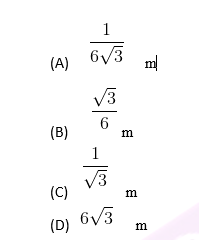Solution: The given situation is explained in the figure below: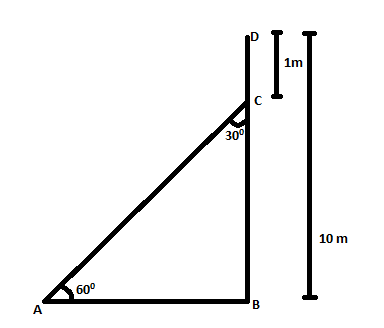DB – DC = CB

⇒BC=9msin60°=BCAC⇒AC=BCsin60°=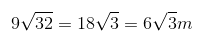∴ The height of the ladder should be 6√3 m.

1. A statue, 2 m tall, stands on the top of a pedestal. From a point on the ground, the angle of elevation of the top of the statue is 60°, and from the same point, the angle of elevation of the top of the pedestal is 45°. Find the height of the pedestal.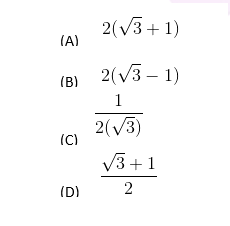Solution: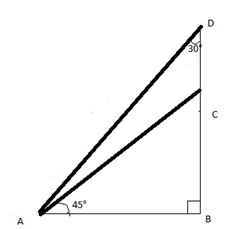CD = 2m

Let BC = x

AB = x (using tan 45∘)

In ΔABD,

BD = AB √3 = x √3

We know that CD = BD – BC = 2m

X(√3 – 1)

X = 2(√3 -1 )

Therefore, the height of the pedestal is 2(√3 -1 ).

1. The angle of elevation of the top of a building from the foot of the tower is 30°, and the angle of elevation of the top of the tower from the foot of the building is 60°. If the tower is 60 m high, find the height of the building.
1. 30 m
2. 40 m
3. 20 m
4. 10 m

Solution: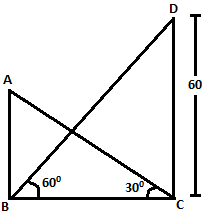The given situation is explained in the figure above.

tan60°=DC/BC⇒BC=DC/tan60°= 60/ √3 = 20 √3 m

tan30°=AB/BC

⇒AB=BC×tan30°=20 √3 × (1/√3 m) = 20 m

Thus, the height of the building is 20 m.

1. A TV tower stands vertically on a bank of a canal, with a height of 10 √3 m. From a point on the other bank directly opposite the tower, the angle of elevation of the top of the tower is 60°. From another point on the line joining this point to the foot of the tower, the angle of elevation of the top of the tower is 30°. Find the distance between the opposite bank of the canal at the point with a 30° angle of elevation.
1. 30 m
2. 20 m
3. 45 m
4. 35 m

Solution: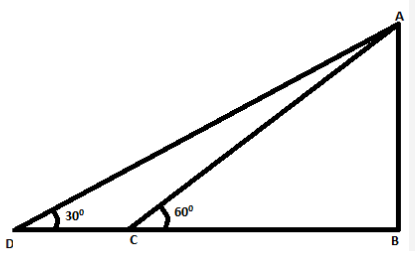The above figure represents the situation given in the question.

tan60°=AB/ BC

⇒AB=BCtan60°= BC √3……….(1)

⇒BC=AB/tan60°=AB/ tan60°

tan30°=AB/BD=AB/ (CD+BC)

⇒DC+BC=AB/tan30°=AB √3

⇒DC=AB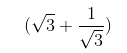⇒DC = 20 m

Hence, the distance between the opposite bank of the canal is 20 m.

1. As observed from the top of a 150 m high lighthouse from the sea level, the angles of depression of the two ships are 30° and 45°. If one ship is exactly behind the other on the same side of the lighthouse, find the distance between the two ships.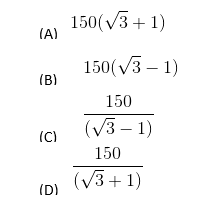Answer: (B) 150 (√3 – 1)

Solution: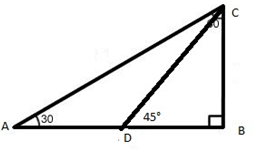Here, the lighthouse, BC = 150 m

In ΔBDC,

BD = BC = 150 m (using tan 45°)

In ΔABC,

AB = BC √3 (using tan 30°)

AB= 150√3

So, distance AD = 150 (√3 – 1)

Hence, the between the two ships is 150 (√3 – 1).

1. An observer of √3m tall is 3 m away from a pole of 2√3 high. What is the angle of elevation of the top of the pole from the observer?
1. 60°
2. 30°
3. 45°
4. 90°

Solution: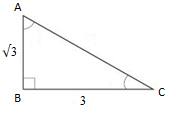Height of the pole that is above man’s height = 2 √3 – √3 = √3 m

Hence, AB = √3 m

BC = 3 m

Hence, tan C = AB/BC =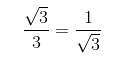⟹ C, angle of elevation = 30°

Therefore, the angle of elevation of the top of the pole from the observer is 30°.

### Introduction

1. The angle of elevation of the top of a tower from a point on the ground, which is 30 m away from the foot of the tower, is 30°. Find the height of the tower.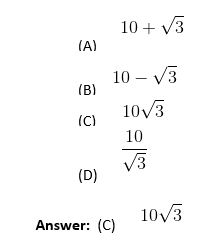Solution: In ΔABC, taking the tangent of ∠C, we have,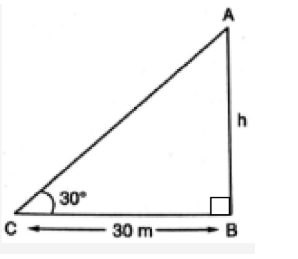tan C=AB/BC

⇒tan 30°=h/30

$$\begin{array}{l}\frac{1}{\sqrt{3}}\end{array}$$
= h/30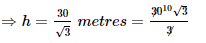=10(1.732)

=17.32m = 10√3 m

Hence, the height of the tower is 10√3 metres.

1. An observer having 1.5 m tall is 28.5 m away from a tower. The angle of elevation of the top of the tower from her eyes is 45°. What is the height of the tower?
1. 20 m
2. 10 m
3. 40 m
4. 30 m

Solution: Let AB be the tower of height h, and CD be the observer of height, 1.5 m at a distance of 28.5 m from tower AB.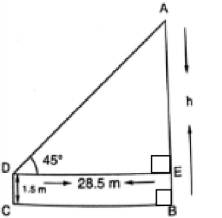In ΔAED, we have

tan45°=h/28.5

⇒1=h/28.5

⇒h=28.5 m

∴ h=AE+BE=AE+DC

= (28.5+1.5) m=30 m

Height of tower = h + 1.5

= 28.5 + 1.5

= 30 m

Hence, the height of the tower is 30 m.

1. An electrician has to repair an electrical fault on a pole of a height of 4 m. He needs to reach a point 1.3 m below the top of the pole to undertake the repair work. What should be the length of the ladder that he should use when inclined at an angle of 60° to the horizontal that would enable him to reach the required position?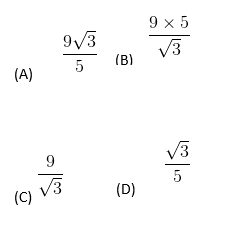Answer: (A)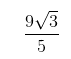Solution: Let AC be the electric pole of height 4 m. Let B be a point 1.3 m below the top A of the pole AC.

Then, BC = AC – AB = (4 – 1.3) m = 2.7 m

Let BD be the ladder inclined at an angle of 60° to the horizontal.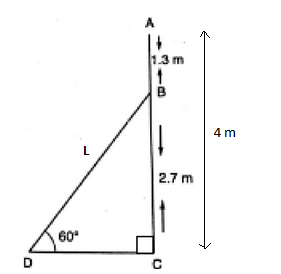In ΔBCD, we have

sin60°=2.7/L.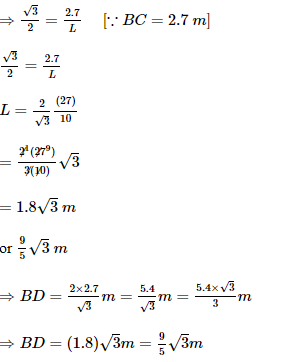Hence, the length of the ladder should bem.

1. A circus artist is climbing a 20 m long rope, which is tightly stretched and tied from the top of a vertical pole to the ground. Find the height of the pole if the angle made by the rope with the ground level is 30°.
1. 10 m
2. 15 m
3. 20 m
4. 35 m

Solution: Let AB be the vertical pole and CA be the 20 m long rope, such that one end is tied from the top of the vertical pole AB and the other end C is tied to a point C on the ground.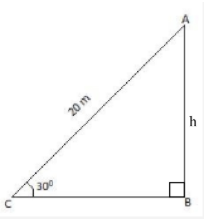In ΔABC, we have

sin 30°=h/20

⇒1/2=h/20

⇒h=10 m

Hence, the height of the pole is 10 m.

### Heights and Distances

1. An observer of 2.25 m tall is 42.75 m away from a chimney. The angle of elevation of the top of the chimney from her eyes is 45°. What is the height of the chimney?
1. 40 m
2. 50 m
3. 45 m
4. 35 m

Solution: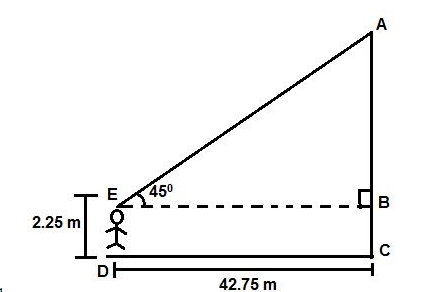The given situation is explained in the figure above:

In triangle ABE,

tan45°=AB/EB

Also, EB=DC

∴ tan45°=AB/ DC

⇒AB=DC × tan 45°

⇒AB=1×42.75

Hence, the height of the chimney = AC = AB + BC

We can observe that BC = ED.

Thus, AC = AB + ED

= 42.75 + 2.25

= 45 m.

Hence, the height of the chimney is 45 m.

1. A tower stands vertically on the ground. From a point on the ground, which is 30 m away from the foot of the tower, the angle of elevation of the top of the tower is found to be 30°. Find the height of the tower.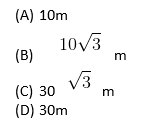Solution: The given situation is explained in the Δ below: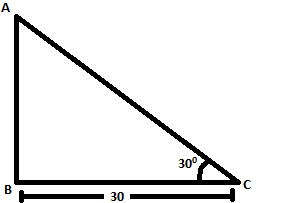Now, tan30°=AB/ BC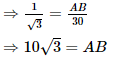∴ The height of the tower is  10√3 m.

1. The angles of depression of the top and the bottom of a 10 m tall building from the top of a multi-storeyed building are 30° and 45°, respectively. Find the height of the multi-storeyed building.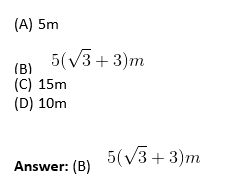Solution: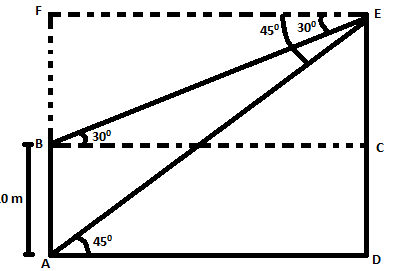The above figure represents the situation aptly.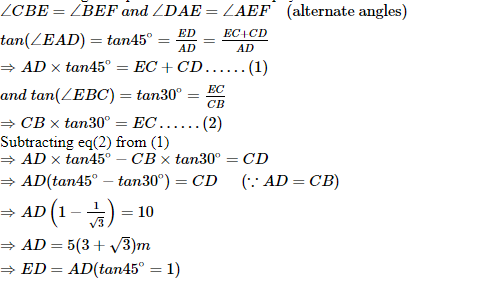(ED is the height of the building)

1. A contractor plans to install two slides for the children to play in a park. For the children below the age of 5 years, she prefers to have a slide whose top is at the height of 2 m and is inclined at an angle of 30° to the ground. What should be the length of the slide?
1. 4
2. 2
3. 1.5
4. 3

Solution: The given situation can be explained using the figure below: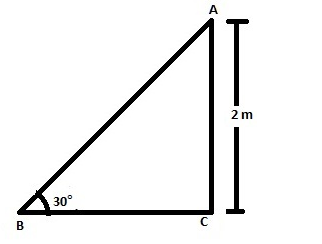In the right-angled triangle ABC,

sin∠ABC=AC/AB=1/2

⇒sin30°=2/AB⇒AB= 2/ (1/2)

⇒AB = 4m

∴ The length of the slide is 4m.

1. A kite is flying at a height of 30 m above the ground. The string attached to the kite is temporarily tied to a point on the ground. The inclination of the string with the ground is 60°. Find the length of the string, assuming that there is no slack in the string.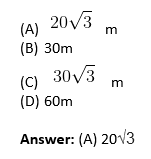Solution: The situation can be explained using the figure below: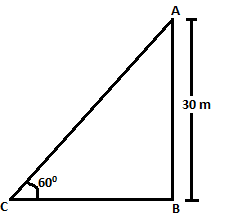In the given right-angled triangle:

sin (∠ACB) =AB/AC

⇒sin60°=AB/AC

⇒AC=AB/sin60°=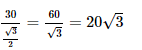∴ The length of the string is 20√3 m

1. A vertical pole of 30 m is fixed on a tower. From a point on the level ground, the angles of elevation of the top and bottom of the pole are 60° and 45°. Find the height of the tower.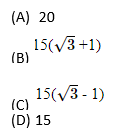Answer: (B) 15 (√3 + 1)

Solution: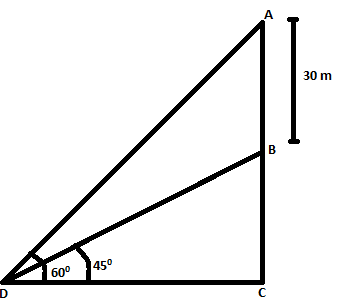The situation can be explained by the figure above.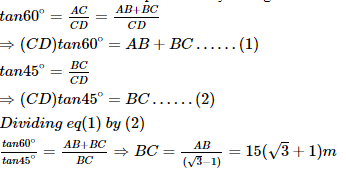Therefore, the height of the tower is 15 (√3 + 1).

1. The value of tan A +sin A=M, and tan A – sin A=N.

The value of (M2−N2) / (MN) 0.5

1. 4
2. 3
3. 2
4. 1

Solution: M2-N2 = (Tan A+ Sin A + Tan A –Sin A) (Tan A +Sin A – Tan A+ Sin A)

M2-N2 =4 tan A sin A

And (MN) 0.5 = (tan2A−sin2A) 0.5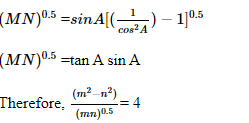1. Two towers, A and B, are standing at some distance apart. From the top of tower A, the angle of depression of the foot of tower B is found to be 30°. From the top of tower B, the angle of depression of the foot of tower A is found to be 60°. If the height of tower B is ‘h’ m, then the height of tower A in terms of ‘h’ is _____ m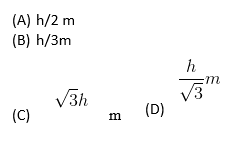Solution: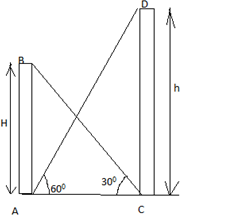Let the height of tower A be = AB = H.

And the height of tower B = CD = h

In triangle ABC,

tan30° = AB/AC = H/AC ……………………………. 1

tan60° = CD/AC = h/AC………………………………….2

Divide 1 by 2

We get tan30°/tan60° = H/h

Therefore, H = h/3 m.

1. A 1.5 m tall boy is standing some distance from a 31.5 m tall building. If he walks ’d’ m towards the building, the angle of elevation of the top of the building changes from 30° to 60°. Find the length of d. (Take √3 = 1.73)
1. 30.15 m
2. 38.33 m
3. 22.91 m
4. 34.55 m

Solution: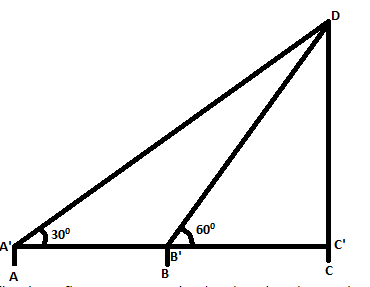The above figure explains the situation given in the question.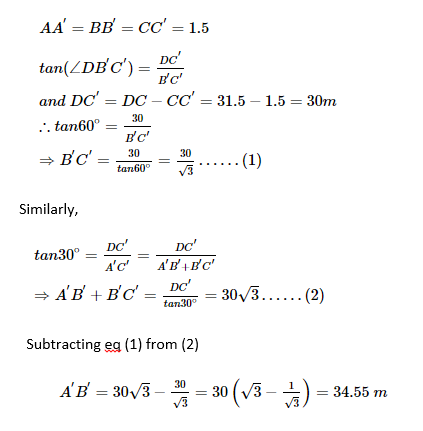∴ The distance moved by the boy, d, is 34.55 m.

1. The angles of depression of two objects from the top of a 100 m hill lying to its east are found to be 45° and 30°. Find the distance between the two objects. (Take √3 = 1.73,)
1. 200 m
2. 150 m
3. 107.5 m
4. 73.2 m

Solution: Let C and D be the objects and CD be the distance between the objects.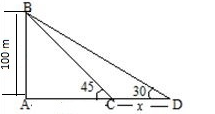In ΔABC, tan 45° = AB/AC

AB=AC=100 m

In ΔABD, tan 30° = AB/AD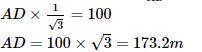Hence, the distance between the two objects is 73.2 metres.

You can access the PDF link to download multiple-choice questions from Chapter 9 – Applications of Trigonometry, categorised as per the topics from which it is taken. Solving these questions will help the students to score better in the board exams because, as per the latest exam pattern, more objective questions are expected to be included in question papers.

### CBSE Class 10 Maths Chapter 9 Extra MCQs

1. If a man standing on a platform 3 metres above the surface of a lake observes a cloud and its reflection in the lake, calculate the angle of elevation of the cloud.

(a) equal to the angle of depression of its reflection.

(b) double to the angle of depression of its reflection

(c) not equal to the angle of depression of its reflection

(d) information insufficient

2. There are two windows in the house. A window of the house is at a height of 1.5 m above the ground, and the other window is 3 m vertically above the lower window. Ram and Shyam are sitting inside the two windows. At an instant, the angle of elevation of a balloon from these windows is observed as 45° and 30°, respectively. Then, what is the height of the balloon from the ground?

(a)  7.269 m

(b) 8.269 m

(c)  7.598 m

(d) 8.598 m

Apart from these MCQs, students can also download other resources at BYJU’S like NCERT Solutions, previous years’ papers, Syllabus, study notes, sample papers, and important questions for all the classes to prepare more efficiently for the exams. Keep learning and stay tuned for further updates on CBSE.